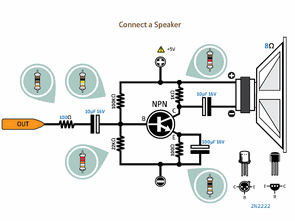ARDUINO EXTERNAL CIRCUIT CONNECTION CHARTS

Arduino transistors, LEDs, motors, MOSFETs, various electronic components, circuit connection diagrams that will be of great use for Arduino projects, Arduino circuits or those who are new to Arduino programming … Circuit diagrams are… Electronics Projects, Arduino External Circuit Connection Charts “arduino projects, “Arduino transistors, LEDs, motors, MOSFETs, various electronic components, circuit connection diagrams that will be of great use for Arduino projects, Arduino circuits or those who are new to Arduino programming … Circuit diagrams are simple to understand Detailed studies on the color codes of the resistors used in Arduino circuit connections ….

ARDUINO CONNECTION DIAGRAM LIST

Arduino Button GND connection, Button 5V connection, Button 12V connection, Built-in pullup, Using potentiometer, Photoresistor, Optocoupler inputs

Arduino Led, Lamp, Dual LEDs, Dual Color LED Connections, Arduino Relay, Mosfet, Buzzer, Sound Amplifier Connections, Arduino Triac, Speaker Connections, Arduino DC Motor, RGB Led Strip Led, Encoder Connections

Bidirectional Voltage Level Converter 3.3V to 5V, Bidirectional Voltage Level Transformer 3.3V to 5V with TTL / CMOS converter, TTL / CMOS converter (6 inputs / outputs) with voltage divider,

Arduino Microphone, Arduino Servo Motor Connections, Arduino Port Replication, 7 Segment Use, Arduino 7 Segment (Common Anode), Multiplexer, LED Array

Arduino LCD HITACHI 44780, Arduino I2C with an LCD HITACHI 44780, Arduino NOKIA LCD (Basic) with 7 segments use with Arduino MAX7221 (Common Anode)

Arduino Unipolar stepper motor drive (Basic 1), Arduino A Bipolar stepper motor drive (Basic 1), Graphical LCD connection via Arduino I2C

Arduino SD Card, Simple 3.3V Power Supply Connection, Arduino Temperature Sensor (LM35), RTC (DS1307), Video, VGA Connection

Digital Temperature Sensor (DS18B20), Simple Rain Sensor with Arduino, AC input, Port Expander (PCF8574xx), Shift (74HC595), Motor Drive (L298), Stepper Drive (L293)

EEPROM connection via I2C, Arduino Distance Sensor, Digital Potentiometer (MCP4161), DAC (MCP4921)

RS485 Node, DMX Controller (Basic), Resistor Color Codes, Ceramic Capacitor Codes, Polyester Film-Mylar Capacitor Code, Push Button Default, Simple Rescue, Rotary Encoder

Arduino Logical Analyzer, Current Sensitivity, Arduino Component Tester (Basic), Simple Transistor Comparison Table

EXAMPLE ARDUINO CONNECTION SCHEMESThis Post / Project can also be found using search terms:

• connection for ckt diagram for stepper motor in advance tech kit
• stepper motor projects using arduino
• ttl connection using plc to arduino circuit